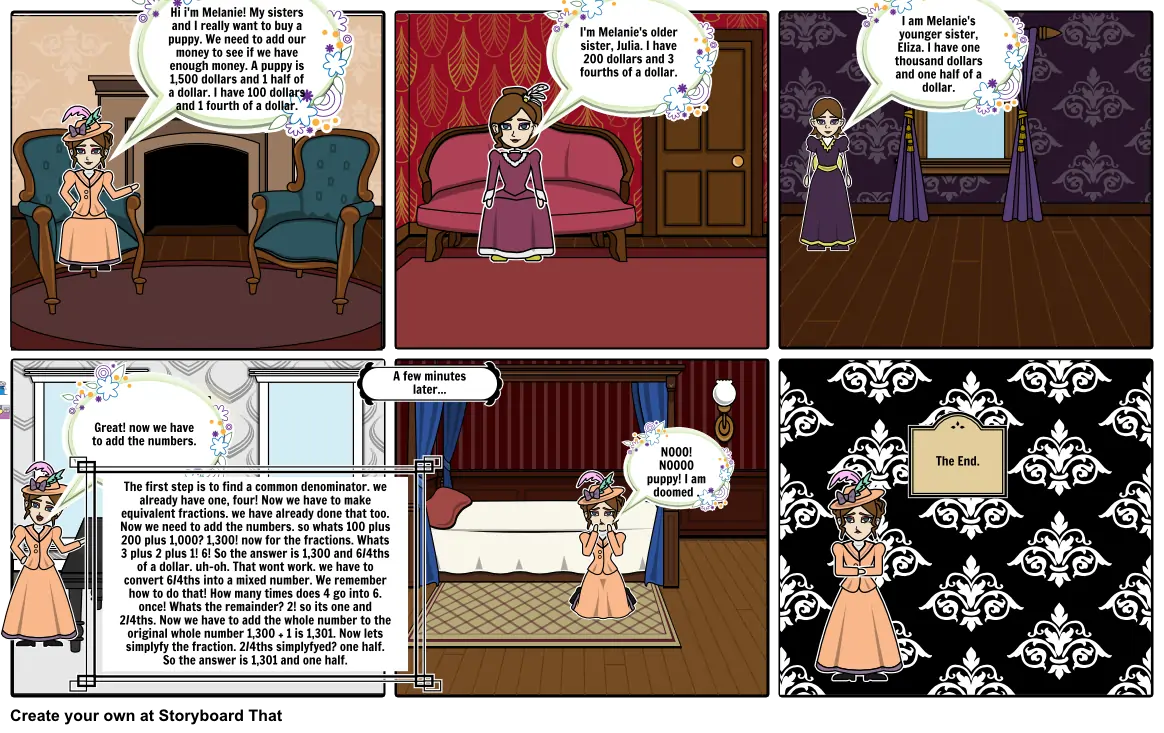# Rich people#### Storyboard Text

• Hi i'm Melanie! My sisters and I really want to buy a puppy. We need to add our money to see if we have enough money. A puppy is 1,500 dollars and 1 half of a dollar. I have 100 dollars and 1 fourth of a dollar.
• I'm Melanie's older sister, Julia. I have 200 dollars and 3 fourths of a dollar.
• I am Melanie's younger sister, Eliza. I have one thousand dollars and one half of a dollar.
• Great! now we have to add the numbers.
• The first step is to find a common denominator. we already have one, four! Now we have to make equivalent fractions. we have already done that too. Now we need to add the numbers. so whats 100 plus 200 plus 1,000? 1,300! now for the fractions. Whats 3 plus 2 plus 1! 6! So the answer is 1,300 and 6/4ths of a dollar. uh-oh. That wont work. we have to convert 6/4ths into a mixed number. We remember how to do that! How many times does 4 go into 6. once! Whats the remainder? 2! so its one and 2/4ths. Now we have to add the whole number to the original whole number 1,300 + 1 is 1,301. Now lets simplyfy the fraction. 2/4ths simplyfyed? one half. So the answer is 1,301 and one half.
• A few minutes later...
• NOOO! NOOOO puppy! I am doomed .
• The End.
##### Over 20 Million Storyboards Created